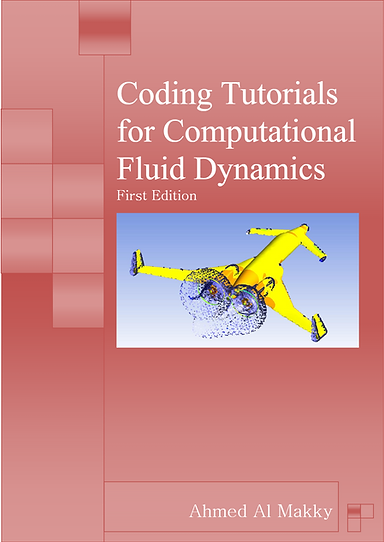# Want to learn CFD Coding!!

## Coding Tutorials

Coding Tutorials for Computational Fluid Dynamics

The tutorials will be focusing on improving the skills of students working in the field of CFD. They will be split for beginners and intermediate students. The main goal of these tutorials is to be as a  step by step guide to building coding skill.  This is achieved by providing examples that are related to the application side of things in the field.  The user will have to work on both  FORTRAN,  MATLAB, and  Excel.

(PDF) Coding Tutorials for Computational Fluid Dynamics. Available from: Research Gate

https://www.researchgate.net/publication/310501995_Coding_Tutorials_for_Computational_Fluid_Dynamics## Theoretical     Resources

### Basic:

J. Anderson Computational Fluid Dynamics

Divided into 4 parts with 12 chapters, Computational Fluid Dynamics: The Basics With Applications covers all that is needed for students to efficiently move on to advanced concepts. Part one introduces readers to the fundamental philosophy of CFD. It also includes a comprehensive discussion on the governing physical equations. Part two is reserved for the basics of numerical discretization and this section also contains problems for students to solve. Part three elaborates on some vital and practical applications of CFD. The last part, categorized as Other Topics, deals with a few complex concepts and discusses the future of CFD. There also is a useful appendix involving the application of Thomas' Algorithm.

The book also illustrates and details various computer graphics used in CFD. As students require a considerable period of time for learning and assimilating the subject before doing successful calculations on their own, the author has included only a few problems to be worked out.### Intermediate

Joel H. Ferziger, Milovan Peric -Computational Methods For Fluid Dynamics

The book shows common roots and basic principles for many different methods. The book also contains a great deal of practical advice for code developers and users, it is designed to be equally useful to beginners and experts. The issues of numerical accuracy, estimation and reduction of numerical errors are dealt with in detail, with many examples. A full-feature user-friendly demo-version of a commercial CFD software has been added, which can be used to compute all flow examples from the book. All computer codes can be accessed from the publishers server on the internet.

https://app.digiboxx.com/public/6362180Applied Computational Fluid Dynamics - SC Gupta

It is hoped that the book is valuable as learning and teaching resources to support CFD courses at undergraduate and postgraduate levels. It intends to bridge the gap between fluid dynamics concepts, the academic CFD literature, and applied industrial practice. A good grip of CFD is possible with good quality codes and with hands-on experience with solutions for aerodynamically featured designs. The book will also be useful to professional engineers at the industry, involved in R&D and design-related work.### Discretization

• Discretization: The word comes from "discrete" and is defined as "constituting a separate thing; individual; distinct; consisting of unconnected distinct parts".

• Discretization is the process by which a closed-form mathematical expression, such as a function or a differential or integral equation involving functions, all of which are viewed as having an infinite continuum of values throughout some domain, is approximated by analogous (but different) expressions which prescribed values only at a finite number of discrete points or volumes in the domain.

Numerical solutions can give answers at only discrete points in the domain, called grid points.

If the PDEs are totally replaced by a system of algebraic equations which can be solved for the values of the flow-field  variables at the discrete points only, in this sense, the original PDEs have been discretized. Moreover, this method of discretization is called the method of finite differences.### Finite Differences:

A finite difference is a mathematical expression of the form f (x + b) − f (x + a). If a finite difference is divided by b − a, one gets a difference quotient. The approximation of derivatives by finite differences plays a central role in finite difference methods for the numerical solution of differential equations, especially boundary value problems.